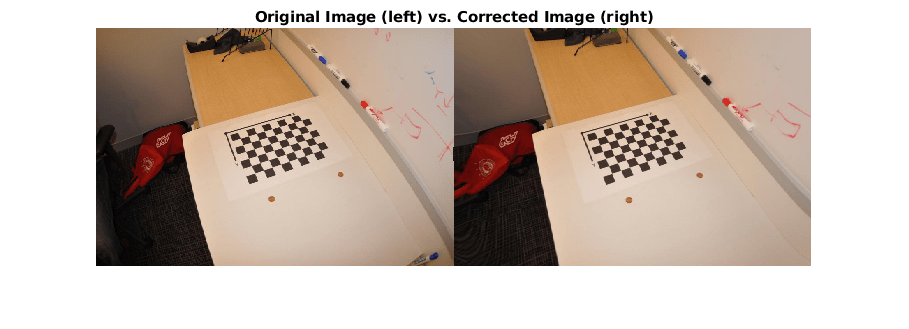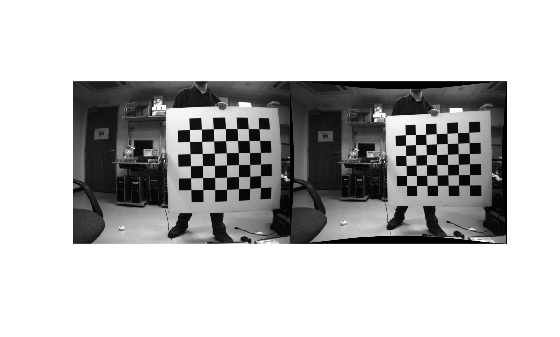cameraIntrinsicsFromOpenCV

Convert camera intrinsic parameters from OpenCV to MATLAB

Description

example

intrinsics = cameraIntrinsicsFromOpenCV(intrinsicMatrix,distortionCoefficients,imageSize) converts the OpenCV intrinsics, specified by the input arguments, into a MATLAB® cameraIntrinsics object intrinsics.

The OpenCV spatial coordinate system specifies the upper-left pixel center at (0,0), whereas the MATLAB spatial coordinate system specifies the pixel center at (1,1). The cameraIntrinsicsFromOpenCV function compensates for this difference by adding 1 to both of the x and y-values for the converted principal point.

OpenCV intrinsics cannot be converted to a MATLAB cameraIntrinsics object when:

• The OpenCV pinhole camera model uses more than five distortion coefficients.

• An OpenCV fisheye model is used.

In these cases, you can recalibrate the stereo camera using the Camera Calibrator app.

Examples

collapse all

Define OpenCV camera intrinsic parameters in the workspace.

intrinsicMatrix = [729.4644    0       570.6455;
0       728.8196  346.0108;
0         0         1  ];
distortionCoefficients = [-0.4262  0.5460  0.0038  -0.0051  -0.6176];

Define the image size returned by the camera.

imageSize = [712 1072];

Convert the intrinsic parameters from OpenCV to MATLAB format.

intrinsics = cameraIntrinsicsFromOpenCV(intrinsicMatrix, ...
distortionCoefficients,imageSize);

filename = fullfile(toolboxdir("vision"),"visiondata","calibration", ...
"mono","image01.jpg");

Undistort the image and diplay the results.

J = undistortImage(I,intrinsics);
imshowpair(I,J,"montage");
title("Original Image (left) vs. Corrected Image (right)");The ROS camera calibration package estimates camera intrinsic parameters using the OpenCV camera calibration tools . After calibrating a camera in ROS, you can import its intrinsic parameters to a YAML file using the camera calibration parser in ROS. To use the calibrated camera with Computer Vision Toolbox™ functions, such as undistortImage, you must read the camera parameters from the YAML file and then convert them into a cameraIntrinsics object using cameraIntrinsicsFromOpenCV.

Note: The cameraIntrinsicsFromOpenCV function supports importing camera intrinsic parameters for only those pinhole camera models that use the ROS plumb-bob distortion model.

Read Camera Intrinsic Parameters from a ROS YAML File

Read the camera parameters stored in cameraParams.yaml using the helper function helperReadYAML.

Create cameraIntrinsics Object Using cameraIntrinsicsFromOpenCV

Use the cameraIntrinsicsFromOpenCV function to create a cameraIntrinsics object from the camera matrix and the distortion coefficients.

imageSize = [intrinsicsParams.image_height intrinsicsParams.image_width];
intrinsicMatrix = intrinsicsParams.camera_matrix;
distortionCoefficients = intrinsicsParams.distortion_coefficients;

intrinsicsObj = cameraIntrinsicsFromOpenCV(intrinsicMatrix,distortionCoefficients,imageSize);

Undistort Image

Use the imported camera intrinsics with undistortImage to undistort an image captured using the calibrated camera.

imageName = fullfile(toolboxdir('vision'),'visiondata','calibration','stereo','left','left01.png');

% Undistort the image.
J = undistortImage(I,intrinsicsObj,'OutputView','full');

% Display the result.
figure
montage({I,J})Supporting Functions

The helperReadYAML function reads the monocular camera parameters from the input YAML file that was exported from ROS.

% helperReadYAML reads a ROS YAML file, filename, and returns a structure
% with these fields: image_width, image_height, camera_name,
% camera_matrix, distortion_model, distortion_coefficients,
% rectification_matrix, and projection_matrix. These fields are stored
% in the YAML file colon separated from their values in different lines.

f = fopen(filename,'r');
stringFields = {'camera_name','distortion_model'};

while ~feof(f)

if isEmptyLine
continue
end

if ~isempty(value)
% Convert all values to numbers except for known string
% fields.
if ~any(contains(name, stringFields))
value = str2num(value); %#ok
end
else
% An empty value in ROS YAML files indicates a matrix in
% upcoming lines. Read the matrix from the upcoming lines.
end

% Store post-processed value.
cameraParams.(name) = value;
end

fclose(f);
end

The helperReadYAMLMatrix function reads the rows, columns and data fields of a matrix in the ROS YAML file.

%   helperReadYAMLMatrix reads a matrix from the ROS YAML file. A matrix in
%   a ROS YAML file has three fields: rows, columns and data. rows and col
%   describe the matrix size. data is a continguous array of the matrix
%   elements in row-major order. This helper function assumes the presence
%   of all three fields of a matrix to return the correct matrix.

numRows = 0;
numCols = 0;
data = [];

% Read numRows, numCols and matrix data.
while ~feof(f)

if isEmptyLine
continue
end

switch name
case 'rows'
numRows = str2num(value); %#ok
case 'cols'
numCols = str2num(value); %#ok
case 'data'
data    = str2num(value); %#ok

% Terminate the while loop as data is the last
% field of a matrix in the ROS YAML file.
break
otherwise
% Terminate the while loop if any other field is
% encountered.
break
end
end

if numel(data) == numRows*numCols
% Reshape the matrix using row-major order.
matrix = reshape(data,[numCols numRows])';
end
end

line = fgetl(f);

% Trim leading and trailing whitespaces.
line = strtrim(line);

if isempty(line) || line(1)=='#'
% Empty line or comment.
name = '';
value = '';
isEmptyLine = true;
else
% Split the line to get name and value.
c = strsplit(line,':');
assert(length(c)==2,'Unexpected file format')

name = c{1};
value = strtrim(c{2}); % Trim leading whitespace.
isEmptyLine = false;
end
end

References

Input Arguments

collapse all

Camera intrinsic matrix from OpenCV, specified as a 3-by-3 matrix of the form:

$\left[\begin{array}{ccc}fx& 0& cx\\ 0& fy& cy\\ 0& 0& 1\end{array}\right]$

where fx and fy are the focal lengths in the x and y-directions, and (cx,cy) is the principal point in OpenCV.

Camera radial and tangential distortion coefficients from OpenCV, specified as a five-element vector of the form [k1 k2 p1 p2 k3]. The values of k1, k2, and k3 describe the radial distortion and p1 and p2 describe the tangential distortion, specified in OpenCV.

Image size, specified as a 2-element vector in the form [mrows,ncols].

Output Arguments

collapse all

Camera intrinsic parameters, returned as a cameraIntrinsics object.

Introduced in R2021b